# How do you find the domain and range of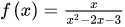?Elsie

Solution:

Concept:The domain of a function is the set of all the values of x for which a function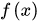is defined and the value which the function takes is the range of that function.

Also, the domain of a functionis equal to the range of its inverse function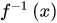and vice-versa.

For a function having rational expression will have domain of all real number except at the roots of the denominator of the rational function.

Calculation:

Step:1 The functionhas denominator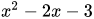.

The roots of the equationcan be calculated using the quadratic formula as shown below:Step:2 Hence the roots of the denominator expression are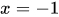and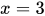.

Step:3 Therefore, the function has domain as the set of all real numbers except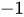and 3, which is written in interval notation as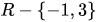.

Step:4 Now, to find the range of the function, first let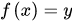and rearrange the function as quadratic expression.For the roots to be real in quadratic equation, it must satisfy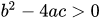.

Substitute the values of a, b and c in the expression.Since for all the values of y the inequality satisfies thus the range of the function is set of all real numbers.

Thus, the domain of the functionisand the range is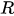.

Answer:The domain of the functionisand the range is.Similar Problems:

Problem 1:What is the domain of a polynomial function.

Solution:

Step:1 The domain of a function is the set of all the values of x for which a functionis defined.

Step:2 For a function having rational expression will have domain of all real number except at the roots of the denominator of the rational function.

Step:3 Since every polynomial function has denominator as a constant value 1 therefore there are no values of x for which the functions is not defined.

Thus, the domain of a polynomial function is the set of all real numbers, which can be written as  .

Answer:The domain of a polynomial function is the set of all real numbers.

Problem 1: What is the domain of a function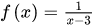.

Solution:

Step:1 The domain of a function is the set of all the values of x for which a functionis defined.

Step:2 For a function having rational expression will have domain of all real number except at the roots of the denominator of the rational function.

Step:3 Since the given function has denominator as a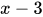therefore for the value of, the functions is not defined.

Step:4 Thus, the domain of a functionis the set of all real numbers except the number 3, which can be written as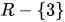.

Answer:The domain of a functionis the set of all real numbers except the number 3, which can be written as.

### Need homework help now?

tutors online

Related Questions
##### (t) in °C of the pot of soup at time t (in The initial temperature of a pot of soup is 80°C. The temperature minutes) satisfies the differential equation dT di T-20 10 (a) Solve the differential equation, and find an explicit expression for the function (). By separating variables, integrating and manipulating arbitrary constants, . We are also told that so that and so . ...
Notes
Me

OR

Don't have an account?

Join OneClass

Access over 10 million pages of study
documents for 1.3 million courses.

Join to view

OR

By registering, I agree to the Terms and Privacy Policies
Just a few more details

So we can recommend you notes for your school.